# Image Quality

Image Quality

The quality of medical images is related to how well they convey specific anatomical or functional information to the interpreting physician such that an accurate diagnosis can be made. Radiological images acquired with ionizing radiation can be made less grainy (i.e., lower noise) by simply turning up the radiation levels used. However, the important diagnostic features of the image may already be clear enough, and the additional radiation dose to the patient is an important concern. Diagnostic medical images, therefore, require thoughtful consideration in which image quality is not necessarily maximized but rather is optimized to perform the specific diagnostic task for which the exam was ordered.

While diagnostic accuracy is the most rigorous measure of image quality, measuring it can be quite difficult, time-consuming, and expensive. In principle, measurement of diagnostic accuracy requires a clinical trial with patient cohorts, well-defined scanning protocols, an adequate sample of trained readers, and procedures for obtaining the ground truth status of the patients. Such studies most certainly have their place in the evaluation of medical imaging methodology, but they also can be a substantial overkill if one is simply deciding between a detector with a pixel pitch of 80 µm and another with 100 µm.

As a result, the vast majority of image quality assessments are not determined from direct measurement of diagnostic accuracy. Instead, they are based on surrogate measures that are linked by assumption to diagnostic accuracy. For example, if we can improve the resolution of a scanner without affecting anything else, then we might assume that diagnostic accuracy improves, or at least does not get any worse. In cases where noise and resolution trade off against each other, image quality measures based on a ratio may be used. This forms the basis for the contrast-to-noise ratio (CNR) and signal-to-noise ratio (SNR) measures. In other situations, a simplified reading procedure is used. These can be used to produce a contrast-detail diagram or a receiver operating characteristic (ROC) curve.

This chapter is intended to elucidate these topics. It is also meant to familiarize the reader with the specific terms used to describe the quality of medical images, and thus the vernacular introduced here is important as well. This chapter, more than most in this book, appeals to mathematics and mathematical concepts, such as convolution and Fourier transforms. Some background on these topics can be found in Appendix G, but to physicians in training, the details of the mathematics should not be considered an impediment to understanding but rather as a general illustration of the concepts. To engineers or image scientists in training, the mathematics in this chapter are a necessary basic look at imaging system analysis.

4.1 SPATIAL RESOLUTION

Spatial resolution describes the level of detail that can be seen on an image. In simplistic terms, the spatial resolution relates to how small of an object can be visualized on a particular imaging system, assuming that other potential limitations of the system
(e.g., object contrast or noise) are not an issue. However, more rigorous mathematical methods based on the spatial frequency spectrum provide a measure of how well the imaging system performs over a continuous range of object dimensions.

Low-resolution images are typically seen as blurry. Objects that should have sharp edges appear to taper off from bright to dark and small objects located near one another appear as one larger object. All of this will generally make identifying subtle signs of disease more difficult. In this section, we will describe some of the reasons why medical images have limited resolution, how blur is modeled as a point spread function (PSF), and how this leads in turn to the concept of a modulation transfer function (MTF).

4.1.1 Physical Mechanisms of Blurring

There are many different mechanisms in medical imaging that cause blurring, and some of these mechanisms will be discussed in length in subsequent chapters. It is important to understand that every step of image generation, acquisition, processing, and display can impact the resolution of an image. This makes resolution a relevant topic of consideration throughout the imaging process.

Most x-ray images are generated by electrons impacting an anode at high velocity. The area in which this happens is called the focal spot of the x-ray tube. The width of the focal spot causes points in the field of view to have a width when they are projected onto a detector. This is called focal-spot blur. Similar phenomena occur in other imaging modalities. In PET imaging, positrons diffuse away from the point of radioactive decay before they annihilate, leading to positron range blurring. In ultrasound imaging, the finite width of a transmission aperture limits the focal width of the resulting pulse leading to aperture blur. These examples show how imaging systems have resolution limitations even before they have imaged anything.

Detectors are also a source of blur in imaging systems. When an x-ray or γ-ray strikes an intensifying screen or other phosphors, it produces a burst of light photons that propagate by optical diffusion though the screen matrix. For thicker screens, the diffusion path toward the screen’s surface is longer, and more lateral diffusion occurs, which results in a broader pattern of light reaching the surface of the screen and consequently more blurring. Furthermore, the digital sampling that occurs in detectors results in the integration of the 2D signal over the surface of the detector element, which can be thought of as detector sampling blur.

It is often the case that image data are processed and displayed in ways that suppress noise. This can be accomplished by any of a number of smoothing operations that employ averaging over multiple detector elements or image pixels. These operations usually involve a trade-off between how much noise is suppressed, and how much blur is added to the imaging system.

Blurring means that image intensity, which should be confined to a specific location, is spread over a region. Various spread functions are used to characterize the impact of physical blurring phenomena in medical imaging systems. A common mathematical approach to modeling spread relies on the idea of convolution.

4.1.2 Convolution

Convolution is an integral calculus procedure that accurately describes mathematically what the blurring process does physically under the assumption that the spread function is not changing with position, a property known as shift-invariance. The convolution process is also an important mathematical component of image reconstruction, and understanding the basics of convolution is essential to a complete
understanding of imaging systems. Below, a basic description of the convolution process is provided, with more details provided in Appendix G.

Convolution in 1D is given by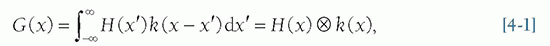where ⊗ is the mathematical symbol for convolution. This equation describes the convolution of an input function, H(x), with a convolution kernel, k(x). Convolution can occur in two or three dimensions as well, and the extension to multidimensional convolution is straightforward: x becomes a two- or three-dimensional vector representing spatial position, and the integral becomes two- or three-dimensional as well.

As a way to better understand the meaning of convolution, let us sidestep the integral calculus of Equation 4-1 and consider a discrete implementation of convolution. In this setting, the input function, H, can be thought of as an array of numbers, or more specifically, a row of pixel values in a digital image. The convolution kernel can be thought of as an array of weights. In this case, we will assume there are a total of five weights each with a value of 0.20 (this is sometimes referred to as a five-element “boxcar” average).

Figure 4-1 shows how these discrete values are combined, resulting in the output column of averaged values, G. In pane A, the first five numbers of H are multiplied by the corresponding elements in the kernel, and these five products are summed, resulting in the first entry in column G. In pane B, the convolution algorithm proceeds by shifting down one element in column H, and the same operation is computed, resulting in the second entry in column G. In pane C, the kernel shifts down another element in the array of numbers, and the same process is followed. This shift, multiply, and add procedure is the discrete implementation of convolution—it is one of the ways this operation can be executed in a computer program. The integral in Equation 4-1 can be thought of as the limit as there are more and more (ever smaller) pixels and weights.

A plot of the values in columns H and G is shown in Figure 4-2, and H, in this case, is the value 50 with randomly generated noise added to each element. The values of G are smoothed relative to H, and that is because the elements of the convolution kernel, in this case, are designed to perform data smoothing—essentially by averaging five adjacent values in column H. Notice that the values of G are generally
closer to the mean value than the original values of H, indicating some suppression of the noise.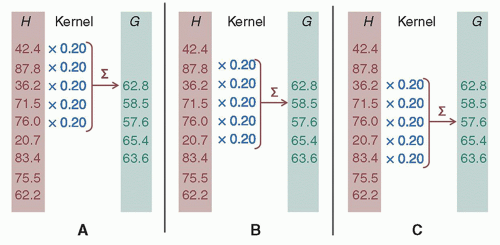▪ FIGURE 4-1 The basic operation of discrete convolution is illustrated. In the three panes (A-C), the input array H is convolved with a five-element boxcar kernel, k, resulting in the output array, G. The different panes show the convolution kernel, and the output value, advancing by one index element at a time. The entire convolution is performed over the complete length of H.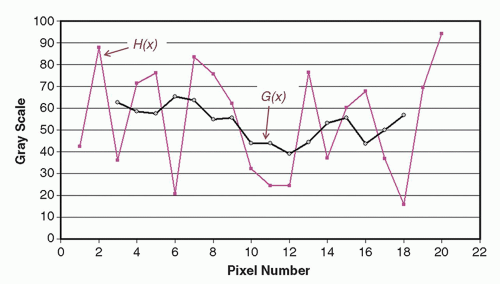▪ FIGURE 4-2 A plot of the noisy input data in H(x), and of the smoothed function G(x), where x is the pixel number (x = 1, 2, 3, …). The first few elements in the data shown on this plot correspond to the data illustrated in Figure 4-1. The input function H(x) is random noise distributed around a mean value of 50. The convolution process with the boxcar average results in substantial smoothing, and this is evident by the much smoother G(x) function that is closer to this mean value.

However, there is generally a price to be paid for smoothing away noise. To illustrate this, consider Figure 4-3. In this case, we will assume that the deviation from the value of 50 is not due to noise. Instead, these points represent some sort of fine structure in the image. Here, the effect of the boxcar average is to blur these points. On the left side of the plot, the single-pixel structure gets turned into a 5-pixel “blob” after this convolution. And the 3-pixel structure on the right side gets turned into a 7-pixel blob that has completely lost the appearance of a valley between two high points. Thus, the boxcar filter spreads the intensity of these fine features over many more pixels and can erase potentially important features. It is also worth noting that the peak intensity of the features has been lowered considerably.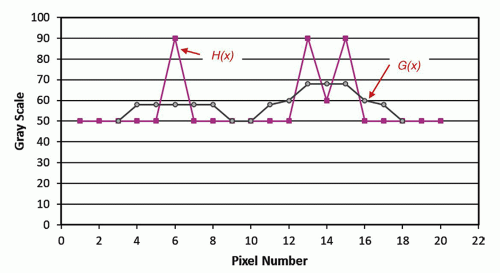▪ FIGURE 4-3 Unlike Figure 4-2, in this case, we see the effect of smoothing on fine image structure (signal). Here the departures from the mean value of 50 are because of fine structure in the image. The boxcar average lowers peak image intensity and spreads it out over a wider region. It completely removes the valley between the two peaks on the left.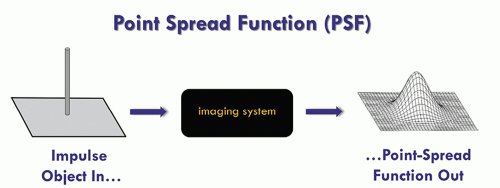▪ FIGURE 4-4 An impulse stimulus to an imaging system is illustrated (left), and the response of the imaging system, the point spread function (PSF), is shown (right). The spread of intensity in the PSF is used to model sources of blur in the image. This PSF is rotationally symmetric.

4.1.3 The Spatial Domain: Spread Functions

In radiology, images vary in size from small spot images acquired in mammography (˜50 mm × 50 mm) to the chest radiograph, which is 350 mm × 430 mm. These images are viewed in the spatial domain, which means that every point (or pixel) in the image represents a position in 2D or 3D space. The spatial domain refers to the two dimensions of a single image or the three dimensions of a volumetric image such as computed tomography (CT) or magnetic resonance images (MRI). Several metrics that are measured in the spatial domain and characterize the spatial resolution of an imaging system are discussed below.

The PSF is the most basic measure of the resolution properties of an imaging system, and it is perhaps the most intuitive as well. A point source (or impulse) is input to the imaging system, and the PSF is (by definition) the response of the imaging system to that point input (Fig. 4-4). The PSF is also called the impulse response function. The PSF is most commonly used for 2D imaging modalities, where it is described in terms of the x and y dimensions of a 2D image, PSF(x, y). The diameter of the “point” input should theoretically be infinitely small, but practically speaking, the diameter of the point input should be smaller than the width of a detector element in the imaging system being evaluated.

To produce a point input on a planar imaging system such as in digital radiography or fluoroscopy, a sheet of attenuating metal such as lead, with a very small hole in it,1 is placed covering the detector, and x-rays are produced. High exposure levels need to be used to deliver a measurable signal, given the tiny hole. For a tomographic system, a small-diameter wire or fiber can be imaged with the wire placed normal to the tomographic plane to be acquired.

An imaging system with the same PSF at all locations in the field of view is called shift-invariant, while a system that has PSFs that vary depending on the position in the field of view is called shift variant (Fig. 4-5). In general, medical imaging systems are considered shift-invariant to a first approximation—even if some small shift variant effects are present. Pixelated digital imaging systems have finite detector elements (dexels), commonly in the shape of a square, and in some cases, the detector element is uniformly sensitive to the signal energy across its surface.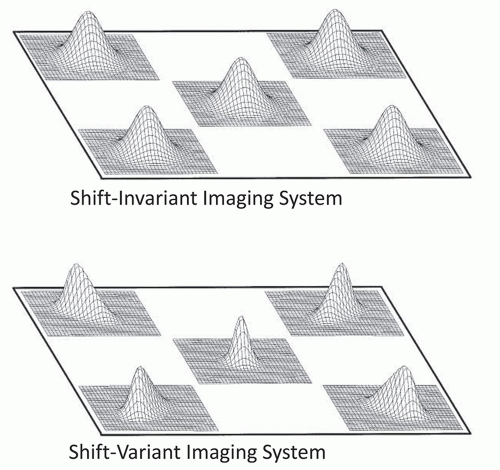▪ FIGURE 4-5 A shift-invariant imaging system is one in which the PSF remains constant over the field of view of the imaging system, as shown in the upper panel. A shift-variant system has a different PSF, depending on the location in the field of view, as shown in the lower panel.

This implies that if there are no other factors that degrade spatial resolution, the digital sampling matrix will impose a PSF, which is in the shape of a square (Fig. 4-6) and where the dimensions of the square are the dimensions of the dexels. The PSF describes the extent of blurring that is introduced by an imaging system, and this blurring is the manifestation of physical events during the image acquisition and subsequent processing of the acquired data.

When an imaging system is stimulated with a signal in the form of a line, the line spread function (LSF) can be evaluated. For a planar imaging system, a slit in some attenuating material can be imaged and would result in a line on the image (Fig. 4-7). Once the line is produced on the image (e.g., parallel to the y-axis), a profile through that line is then measured perpendicular to the line (i.e., along the x-axis). The profile is a measure of grayscale as a function of position. Once this profile is normalized such that the area is unity, it becomes the LSF(x).

From the perspective of making measurements, the LSF has advantages over the PSF. First of all, a slit will generally pass more x-ray photons than a small hole, so the measurement does not require turning up the x-ray intensity as high. There are also some tricks that can be played with the orientation of the slit that allows for very fine sampling of the LSF. We will discuss this topic shortly. However, there are some disadvantages too. The LSF is a somewhat indirect measurement of spread, and it requires some analysis to relate it to the PSF. Additionally, the LSF only makes measurements at the orientation of the slit. If the underlying PSF is asymmetric, the LSF will need to
be acquired at multiple orientations to capture the asymmetry. For digital x-ray imaging systems that have a linear response to x-rays such as digital radiography, the profile can be easily determined from the digital image using appropriate software. Profiles from ultrasound and MR images can also be used to obtain an LSF in those modalities, as long as the signal is converted linearly into grayscale on the images.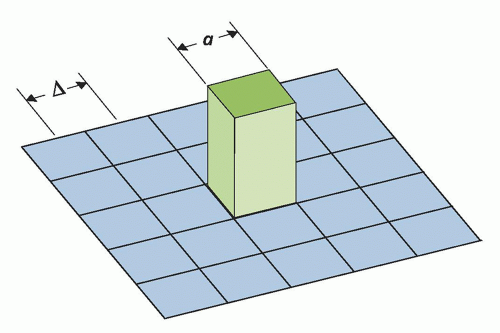▪ FIGURE 4-6 A 2D “RECT” function is shown (see Appendix G), illustrating the PSF of a digital imaging system with square detector elements of width a, in the absence of any other blurring phenomenon. This PSF is the best possible for a digital imaging system.

For a system with good spatial resolution, the LSF will be quite thin, and thus on the measured profile, the LSF may only be a few pixels wide. Consequently, the LSF measurement may suffer from coarse pixel sampling in the image. One way to avoid this is to place the slit at a small angle (e.g., 2° to 8°) to the detector element columns during the physical measurement procedure. Then, instead of taking one profile through the slit image, several profiles are taken at different locations vertically (Fig. 4-8) and the data are synthesized into a presampled LSF. Due to the angulation of the slit, the profiles taken at different vertical positions intersect the slit image at different horizontal displacements through the pixel width—this allows the LSF to be synthesized with sub-pixel spacing intervals, and a better sampled LSF measurement results.

In some situations, PSF and LSF measurements are not ideally suited for a specific imaging application, where the edge spread function (ESF) can be measured. Instead of stimulating the imaging system with a slit image as with the LSF, a sharp edge is presented. The edge gradient that results in the image can then be used to measure the ESF(x). The ESF is particularly useful when the spatial distribution characteristics of glare or scatter phenomenon are the subject of interest—since a large fraction of the field of view is stimulated, low-amplitude effects such as glare or scatter (or both) become appreciable enough in amplitude to be measurable. By comparison, exposure to the tiny area of the detector receiving signal in PSF or LSF measurements would not be sufficient to cause enough optical glare or x-ray scatter to be measurable. A sharp edge is also less expensive to manufacture than a point or slit phantom,
and even spherical test objects can be used to measure the ESF, which is useful when characterization of the spatial resolution in three dimensions is desired.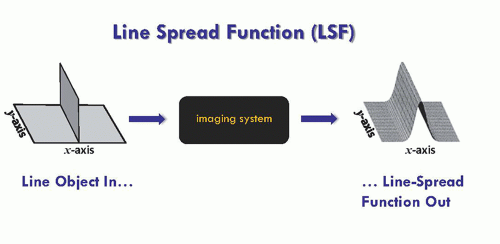▪ FIGURE 4-7 The line impulse function that is input to the imaging system (by imaging a slit for example) leads to an image that has the line-spread function when a profile along the x-axis is taken.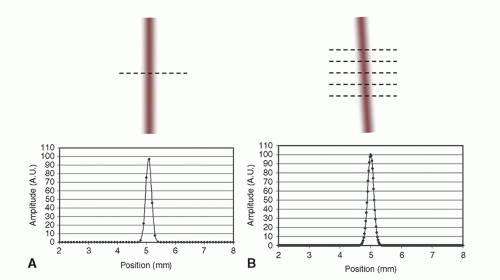▪ FIGURE 4-8 A. The conventional approach to measuring the LSF involves the acquisition of a slit image along the y-axis, and a profile (grayscale versus position) across it (in the x-axis) is evaluated. This approach is legitimate but is limited by the sampling pitch of the detector. B. An alternative approach to computing the LSF was proposed by Fujita. Here, the slit image is acquired at a slight angle (˜5°) relative to the y-axis. At any row along the vertical slit, a single LSF can be measured. However, because of the angle, a composite LSF can be synthesized by combining the LSFs from a number of rows in the image. The angle of the slit creates a small differential shift of the LSF from row to row—this can be exploited to synthesize an LSF with much better sampling than the pixel pitch. This oversampled LSF is called the presampled LSF.

Examples of all three spatial domain spread functions are shown in Figure 4-9.

There are mathematical relationships between the spatial domain spread functions PSF, LSF, and ESF. The specific mathematical relationships are described
here, but the take-home message is that under most circumstances, given a measurement of one of the spread functions, the others can be computed. This feature adds to the flexibility of using the most appropriate measurement method for characterizing an imaging system. These statements presume a rotationally symmetric PSF, but in general, that is the case in many (but not all) medical imaging systems.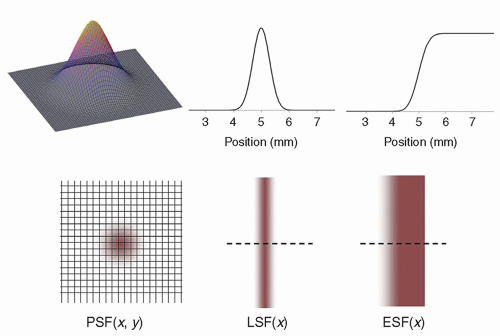▪ FIGURE 4-9 The three basic spread functions in the spatial domain are shown—the PSF(x, y) is a 2D spread function. The LSF and ESF are both 1D spread functions. There is a mathematical relationship between the three spread functions, as discussed in the text.

The LSF and ESF are related to the PSF by the convolution equation. The LSF can be computed by convolving the PSF with an impulse function that is spread over a line2: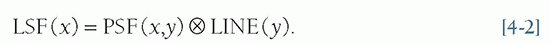Because a line is purely a 1D function, the convolution shown in Equation 4-2 reduces to a simple integral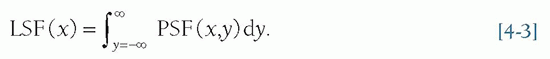Convolution of the PSF with an edge3 results in the ESF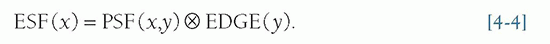In addition to the above relationships, the LSF and ESF are related as well. The ESF is the integral of the LSF, and this implies that the LSF is the derivative of the ESF,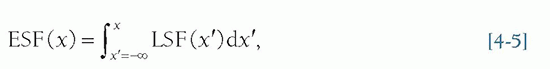where x‘ is a variable of integration.

One can also compute the LSF from the ESF, and the PSF can be computed from the LSF; however, the assumption of rotational symmetry with the PSF is necessary unless multiple orientations of the LSF are used.

4.1.4 The Frequency Domain: Modulation Transfer Function

The PSF, LSF, and ESF are apt descriptions of the resolution properties of an imaging system in the spatial domain. Another useful way to express the resolution of an imaging system is to make use of the spatial frequency domain. If a sine wave has a period of P (the length it takes to complete a full cycle of the wave), then the spatial frequency of the wave is f = 1/P. As this relationship would suggest, a sine wave with a larger period has a smaller spatial frequency and vice versa.

The frequency domain is accessed through the Fourier Transform, which decomposes a signal into sine waves that, when summed, replicate that signal. Once a spatial domain signal is Fourier transformed, the results are considered to be in the frequency domain. The Fourier Transform is a powerful theoretical tool for mathematical analysis of imaging systems, but here we will mostly focus on interpreting the results of this analysis. A more detailed mathematical description of the Fourier Transform can be found in Appendix G.

In Figure 4-10, let the solid black line be a trace of grayscale as a function of position across an image. Nineteenth-century French mathematician Joseph Fourier developed a method for decomposing a function such as this grayscale profile into the
sum of a number of sine waves. Each sine wave has three parameters that characterize its shape: amplitude (a), frequency (f), and phase (ψ), where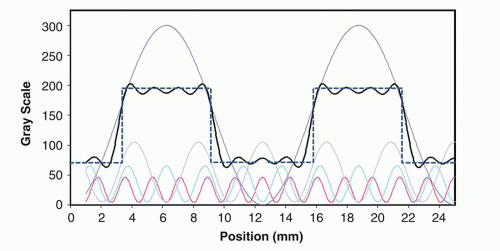▪ FIGURE 4-10 The basic concept of Fourier analysis is illustrated. The solid black lines are the sum of the four sets of sine waves (shown towards the bottom of the plot) and approximate the two RECT functions (dashed lines). Only four sine waves are summed here, but with a more complete set, the RECT functions could be almost perfectly matched. The Fourier transform breaks any arbitrary signal down into the sum of a set of sine waves of different phase, frequency, and amplitude.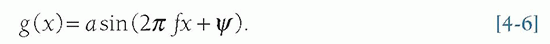Figure 4-10 illustrates the sum of four different sine waves (solid black line), which approximates the shape of two rectangular functions (dashed lines). Only four sine waves were used in this figure for clarity; however, if more sine waves (i.e., a more complete Fourier spectrum) were used, the shape of the two rectangular functions could in principle be matched.

The Fourier transform, FT[ ], converts a spatial signal into the frequency domain, while the inverse Fourier transform, FT-1[], converts the frequency domain signal back to the spatial domain. An important result of linear systems theory is that convolution is equivalent to multiplication in the spatial frequency domain. Referring back to Equation 4-1, the function G(x) can be alternatively computed as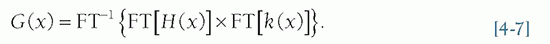Equation 4-7 computes the same function G(x) as in Equation 4-1, but compared to the convolution procedure, in most cases, the Fourier transform computation runs faster on a computer. Therefore, image processing methods that employ convolution filtration procedures such as in CT are often performed in the frequency domain for computational speed. Indeed, the kernel used in CT is often described in the frequency domain (descriptors such as ramp, Shepp-Logan, bone kernel, B41), so it is more common to discuss the shape of the kernel in the frequency domain (i.e., FT[k(x)]) rather than in the spatial domain (i.e., k(x)), in the parlance of clinical CT. Inverse Fourier transforms are used in MRI to convert the received time-domain signal into a spatial signal. Fourier transforms are also used in ultrasound imaging and Doppler systems. All in all, Fourier computations are a routine part of the signal and image processing components of medical imaging systems.

The Modulation Transfer Function

Conceptual Description

Imagine that it is possible to stimulate an imaging system spatially with a pure sinusoidal waveform, as illustrated in Figure 4-11A. The system will detect the incoming sinusoidal signal at frequency f, and as long as the frequency is not too high (relative
to the Nyquist frequency, discussed below), it will produce an image at that same frequency but in most cases with reduced contrast (Fig. 4-11A, right side). The loss of contrast is the result of resolution losses (i.e., blurring) in the imaging system, and can also be thought of as a modulation of the amplitude of the input sinusoid. For input signals with frequencies of 1, 2, and 4 cycles/mm (shown in Fig. 4-11A), the measured signals after passing through the imaging system were 87%, 56%, and 13% of the inputs, respectively. For any one of these three frequencies measured individually,
if the Fourier transform was computed on the recorded signal, the result would be a peak at the corresponding frequency. Three such peaks are shown in Figure 4-11B, representing three individually acquired (and then Fourier transformed) signals. The amplitude of the peak at each frequency reflects the contrast transfer (retained) at that frequency, with contrast losses due to resolution limitations in the system.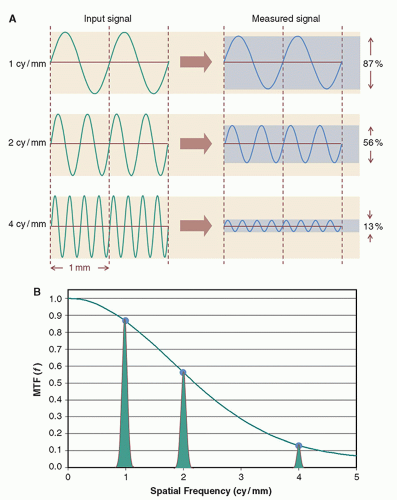▪ FIGURE 4-11 A. Sinusoidal input signals incident on a detector (intensity as a function of position) at three different frequencies are shown as input functions (left). The signals measured by the imaging system are shown on the right. The frequency is the same as the inputs in all cases, but the amplitude of the measured signal is reduced compared to that of the input signal. This reduction in amplitude is a result of resolution losses in the imaging system, which are greater with signals of higher frequencies. For the input at 1 cycle/mm, the original 100% amplitude was attenuated to 87%, and with the 2- and 4-cycle/mm input functions, the resulting signal amplitudes were reduced to 56% and 13%, respectively. B. This figure shows the amplitude reduction as a function of spatial frequency shown in A. At 1 cycle/mm, the system reduced the contrast to 87% of the input. For 2 and 4 mm-1, the signal was modulated as shown. This plot shows the MTF, which illustrates the spatial resolution of an imaging system as a function of the spatial frequency of the input signal.

Interestingly, due to the characteristics of the Fourier transform, the three sinusoidal input waves shown in Figure 4-11A could be acquired simultaneously by the detector system, and the Fourier Transform could separate the individual frequencies and produce the three peaks at F = 1, 2, and 4 cycles/mm shown in Figure 4-11B. Indeed, if an input signal contained more numerous sinusoidal waves (10, 50, 100, …) than the three shown in the figure, the Fourier transform would still be able to separate these frequencies and convey their respective amplitudes from the recorded signal, ultimately resulting in the full, smooth MTF curve shown in Figure 4-11B. Thus the MTF shows how much signal passes through an imaging system as a function of the spatial frequency of the signal.

Limiting Resolution

The MTF gives a rich description of spatial resolution as a function of frequency, and it is the accepted standard for the mathematical characterization of spatial resolution. However, it is sometimes useful to have a single numerical value that characterizes the resolution limit of an imaging system. The limiting spatial resolution is often considered to be the frequency at which the MTF crosses the 10% level (see Fig. 4-12), or some other agreed-upon and specified level.

Nyquist Frequency

Let’s look at an example of an imaging system where the center-to-center spacing (pitch) between each detector element (dexel) is Δ (in mm). In the corresponding image, it would take two adjacent pixels to display a full cycle of a sine wave (Fig. 4-13)—one pixel for the upward lobe of the sine wave, and the other for the downward lobe. This wave is the highest frequency that can be accurately measured on the imaging system. The period of this sine wave is 2Δ, and the corresponding spatial frequency is FN = 1/2Δ. This frequency is called the Nyquist frequency (FN), and it sets an upper bound on the spatial frequency that can be detected for a digital
detector system with detector pitch Δ. For example, for Δ 0.05 mm, FN = 10 cycles/mm, and for Δ = 0.25 mm, FN = 2.0 cycles/mm.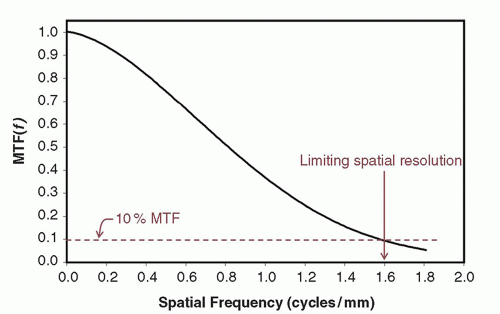▪ FIGURE 4-12 The limiting spatial resolution is the spatial frequency at which the amplitude of the MTF decreases to some agreed-upon level. Here the limiting spatial resolution is shown at 10% modulation, and the limiting spatial resolution is 1.6 cycles/mm.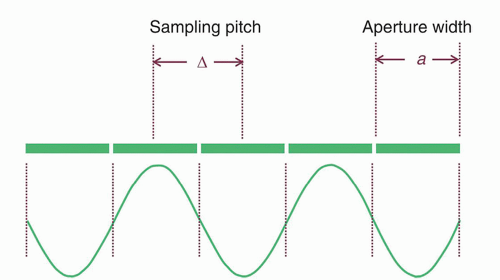▪ FIGURE 4-13 This figure illustrates a side view of detector elements in a hypothetical detector panel. Detectors whose center-to-center spacing (pitch) is about equal to the detector width are very common, and these represent contiguous detector elements. The sampling pitch affects aliasing in the image, while the aperture width of the detector element influences the spatial resolution (the LSF and MTF). A sine wave (shown) where each period just matches the width of two detector elements is the highest frequency sine wave that can be resolved with these detectors due to their sampling pitch.

If a sinusoidal signal greater than the Nyquist frequency were to be incident upon the detector system, its true frequency would not be recorded, but rather it would be aliased. Aliasing occurs when frequencies higher than the Nyquist frequency are imaged (Fig. 4-14). The frequency that is recorded is lower than the incident frequency, and indeed the recorded frequency appears to wrap around the Nyquist frequency. For example, for a system with Δ = 0.100 mm, and thus FN = 5.0 cycles/mm, sinusoidal inputs of 2, 3, and 4 cycles/mm are recorded accurately (along the frequency axis) because they obey the Nyquist Criterion. Since the input functions are single frequencies, the Fourier transform appears as a spike at that frequency, as shown in Figure 4-15. For sinusoidal inputs with frequencies greater than the Nyquist frequency, the signal wraps around the Nyquist frequency—a frequency of 6 cycles/mm (FN + 1) is recorded as 4 cycles/mm (FN – 1), and a frequency of 7 cycles/mm (FN + 2) is recorded as 3 cycles/mm (FN – 2), and so on. This is seen in Figure 4-15 as well. Aliasing is visible when there is a periodic pattern that is imaged, such as an x-ray antiscatter grid, and aliasing appears visually in many cases as a Moiré pattern.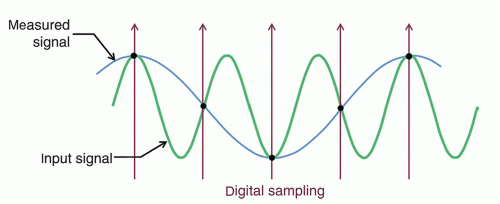▪ FIGURE 4-14 The concept of aliasing is illustrated. The input sine wave (green) is sampled at each of the arrows, but the Nyquist criterion is violated here because the input sine wave frequency is higher than the Nyquist frequency. Thus, the sampled image data will be aliased and have the appearance of a lower frequency sine wave in the measured signal.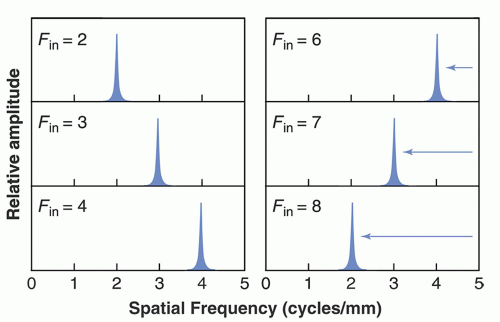▪ FIGURE 4-15 For a single-frequency sinusoidal input function to an imaging system (sinusoidally varying intensity versus position), the Fourier transform of the image results in an impulse at the measured frequency. The Nyquist frequency in this example is 5 cycles/mm. For the three input frequencies in the left panel, all are below the Nyquist frequency and obey the Nyquist criterion, and the measured (recorded) frequencies are exactly what was input into the imaging system. On the right panel, the input frequencies were higher than the Nyquist frequency, and the recorded frequencies in all cases were aliased—they wrapped around the Nyquist frequency—that is, the measured frequencies were lower than the Nyquist frequency by the same amount by which the input frequencies exceeded the Nyquist frequency.

The Presampled MTF

Aliasing can pose limitations on the measurement of the MTF, and the finite size of the pixels in an image can cause sampling problems with the measured LSF that is used to compute the MTF (Eq. 4-3). Figure 4-8 shows the angled slit method for determining the presampled LSF, and this provides a methodology for computing the so-called presampled MTF, which represents blurring processes up to the point at which the signal is sampled. Using a single line perpendicular to the slit image (Fig. 4-8A), the sampling pitch of the LSF measurements is Δ, and the maximum frequency that can be computed for the MTF is then 1/2Δ. However, for many medical imaging systems, it is quite possible that the MTF has non-zero amplitude beyond the Nyquist limit of FN = 1/2Δ.

In order to measure the presampled MTF, the angled-slit method is used to synthesize the presampled LSF. By using a slight angle between the long axis of the slit and the columns of detector elements in the physical measurement of the LSF, different (nearly) normal lines can be sampled (Fig. 4-8B). The LSF computed from each individual line is limited by the Δ sampling pitch, but multiple lines of data can be used to synthesize an LSF, which has much better sampling than Δ. Indeed, oversampling can be performed by a factor of 5 or 10 (etc.), depending on the measurement procedure, the slit-angle relative to the (x, y) matrix, and how long the slit is. The details of how the presampled LSF is computed are beyond the scope of the current discussion; however, by decreasing the sampling pitch from Δ to, for example, Δ/5, the Nyquist limit goes from FN to 5FN, which is often sufficient to accurately measure the presampled MTF.

Field Measurements of Resolution Using Resolution Templates

Spatial resolution should be monitored on a routine basis for many imaging modalities. However, measuring the LSF or the MTF is more detailed than necessary for routine quality assurance purposes. For most clinical imaging systems, the evaluation of spatial resolution using resolution test phantoms is adequate for routine quality
assurance purposes. The test phantoms are usually line-pair phantoms (Fig. 4-16) or star patterns. The test phantoms are imaged, and the images are viewed to estimate the limiting spatial resolution of the imaging system. This is typically reported as line-pairs per mm, which is synonymous with spatial frequency. There is a degree of subjectivity in such an evaluation, but in general, viewers will agree within acceptable limits. These measurements are routinely performed on fluoroscopic equipment, radiographic systems, nuclear cameras, and in CT.Get Clinical Tree app for offline access